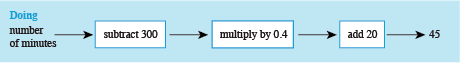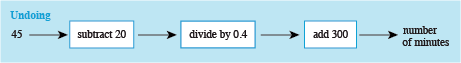Science, Maths & Technology

### Become an OU studentSucceed with maths: part 2

Start this free course now. Just create an account and sign in. Enrol and complete the course for a free statement of participation or digital badge if available.

# 4.1 Time formula

There is just one last activity for you to complete now before moving on to a different subject area. This will give you another opportunity to rearrange a word formula but with a few more elements to consider.

## Activity 9 How many minutes for chatting?

Timing: Allow approximately 5 minutes

This was the formula for the monthly cost in £ of a mobile phone that was used in week 3:

The owner wishes to stick to a monthly budget of £45. To do this they need to know the maximum number of minutes they can use, so that they don’t exceed the budget. Use doing and undoing diagrams to work the maximum number of minutes. Substitute ‘Total monthly cost in £’ for £45.

See if you can then write a word formula that will allow the number of minutes to be calculated for any budget.

Here is the doing diagram:Figure 15 Doing diagram for £45 monthly budget

Undoing each step in turn gives the following undoing diagram.Figure 16 Undoing diagram for number of minutes

Applying these operations in turn gives:

• Subtracting 20 gives:
• Dividing by 0.4 gives:

So, 362 minutes can be used for a monthly budget of £45.

Now all you need to do to provide a word formula for any monthly budget is to change from £45 back to ‘Total monthly cost in £’.

So the word formula will be:

or

Mathematicians prefer the second way of showing this formula just because it looks much neater – otherwise there is no difference between them.

The last part of the activity was more challenging than our other examples, so well done for having a go at it. What you may not realise is that during these last few sections you have been taking your first steps into the wonderful world of algebra. This is one of the fundamental tools of most maths, science and technology, so if you continue into university level study in any of these areas you will certainly find these skills useful. You won’t be using doing and undoing diagrams but techniques used for rearranging formulas (and equations) will be just the same – looking for what ‘undoes’ each operation.

In the next section you are going to look at inequalities. This is the name mathematicians use for expressions that use notation meaning, for example ‘greater than’ and ‘less than’. You have actually come across three examples of these in this week’s and last week’s study, as you will discover when you move on.